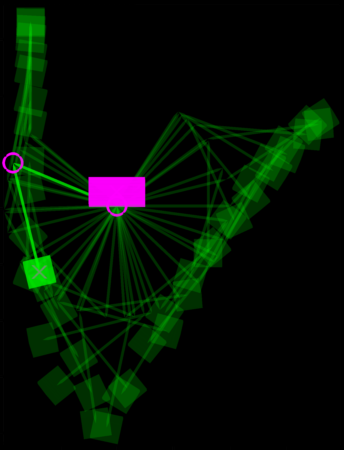# Matlab Code Samples

Both my research work at Cornell and my current spacecraft engineering work involve a fair amount of programming in Matlab. I have some code samples from my Cornell career available here as an example of some of the programs I have produced and because they might be useful to someone out there. The zip files should be extracted to a folder in the Matlab path. Important note: I make these m-files available without any guarantees or support. Documentation may be limited.

## QuIRK Multibody Dynamics Package

Compatibility: Matlab 2009+

QuIRK is an interactive Matlab command line interface for constructing systems of rigid bodies and simple joint constraints, solving the equations of motion of those systems when subject to various force expressions, displaying and animating solved systems, and extracting information about the state history and energetics of those systems. I developed it as a tool for simulating the dynamics of reconfigurable space systems and exploring the possible configurations a system can reach through kinematic reconfiguration control. It is based on quaternion attitude representations of bodies for full generality, and joint constraints are written in terms of the second derivative of body quaternions. Hence: Quaternion-state Interface for Rigid-body Kinetics.Trajectory of a double pendulum in gravity, solved and animated by QuIRK functions.

30-second solution of a double pendulum in gravity by QuIRK functions.

I maintained a site for QuIRK downloads and documentation on my research group's web site.

## Graph Data Structure

Compatibility: Matlab 2009+

In the course of my research, I needed a way to represent a graph structure and perform simple graph operations, such as generating a path between two vertices of the graph. I went back to my computer science notes and wrote these three classes (node, edge, and graph) to take care of that for me in an object-oriented way.

## Orbit Visualization Class

Compatibility: Matlab 2008+

This class defines an "orbit" object by user inputs of Keplerian orbital elements, or from a small library of predefined orbits of planets and moons. Users can alter the orbital elements of an orbit object from the command line using "set" and "get" methods. The "plot" command will produce a visualization of the orbital ellipse, including a marker at the current anomaly of the orbiting body. Note that while changing the anomalies of an orbit object will move the marker along the orbital track according to a low-order expansion of Kepler's Equation, I defined this class primarily for visualization and not for orbital mechanics simulations.

For a quick and entertaining demonstration of the class' capabilities, try out this command sequence:

for t = 1:0.1:100

plot(orbit('mercury', 't', t), orbit('venus', 't', t), ...

orbit('earth', 't', t), orbit('mars', 't', t), ...

orbit('jupiter', 't', t), orbit('saturn', 't', t), ...

orbit('uranus', 't', t), orbit('neptune', 't', t), ...

orbit('pluto', 't', t))

axis equal

pause(0.01)

end

## Flux-Pinning Graphics Toolbox

Compatibility: Matlab 2007+

This toolbox contains functions that generate and manipulate Matlab graphics primitives. I use it to generate a number of the graphics I produce in the course of my research. It behaves in a "psuedo-object-oriented" manner in that there are functions to move, rotate, or change the properties of the graphics objects, which include cubes, cylinders, spacecraft modules, and magnetic field lines.

Example of a movie created with my flux-pinning graphics functions.

Content of this site copyright Joseph Shoer unless otherwise noted.
This is the personal web space of Joseph Shoer and any opinions or conclusions presented here are his alone.